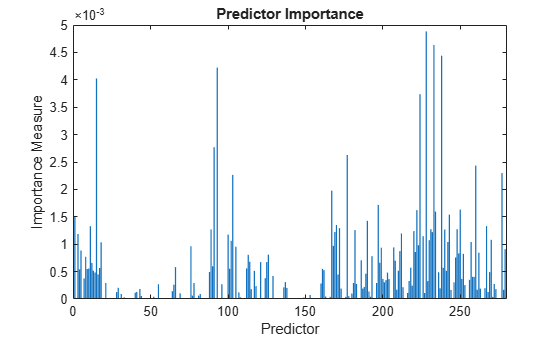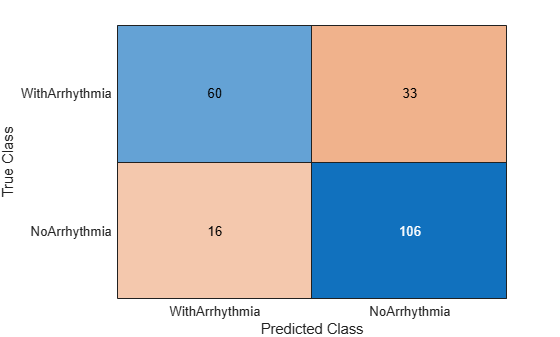# loss

Classification error

## Syntax

```L = loss(ens,tbl,ResponseVarName) L = loss(ens,tbl,Y) L = loss(ens,X,Y) L = loss(___,Name,Value) ```

## Description

`L = loss(ens,tbl,ResponseVarName)` returns the classification error for ensemble `ens` computed using table of predictors `tbl` and true class labels `tbl.ResponseVarName`.

`L = loss(ens,tbl,Y)` returns the classification error for ensemble `ens` computed using table of predictors `tbl` and true class labels `Y`.

`L = loss(ens,X,Y)` returns the classification error for ensemble `ens` computed using matrix of predictors `X` and true class labels `Y`.

`L = loss(___,Name,Value)` computes classification error with additional options specified by one or more `Name,Value` pair arguments, using any of the previous syntaxes.

When computing the loss, `loss` normalizes the class probabilities in `ResponseVarName` or `Y` to the class probabilities used for training, stored in the `Prior` property of `ens`.

Note

If the predictor data `X` or the predictor variables in `tbl` contain any missing values, the `loss` function can return NaN. For more details, see loss can return NaN for predictor data with missing values.

## Input Arguments

 `ens` Classification ensemble created with `fitcensemble`, or a compact classification ensemble created with `compact`. `tbl` Sample data, specified as a table. Each row of `tbl` corresponds to one observation, and each column corresponds to one predictor variable. `tbl` must contain all of the predictors used to train the model. Multicolumn variables and cell arrays other than cell arrays of character vectors are not allowed. If you trained `ens` using sample data contained in a table, then the input data for this method must also be in a table. `ResponseVarName` Response variable name, specified as the name of a variable in `tbl`. You must specify `ResponseVarName` as a character vector or string scalar. For example, if the response variable `Y` is stored as `tbl.Y`, then specify it as `'Y'`. Otherwise, the software treats all columns of `tbl`, including `Y`, as predictors when training the model. `X` Matrix of data to classify. Each row of `X` represents one observation, and each column represents one predictor. `X` must have the same number of columns as the data used to train `ens`. `X` should have the same number of rows as the number of elements in `Y`. If you trained `ens` using sample data contained in a matrix, then the input data for this method must also be in a matrix. `Y` Class labels of observations in `tbl` or `X`. `Y` should be of the same type as the classification used to train `ens`, and its number of elements should equal the number of rows of `tbl` or `X`.

### Name-Value Arguments

Specify optional pairs of arguments as `Name1=Value1,...,NameN=ValueN`, where `Name` is the argument name and `Value` is the corresponding value. Name-value arguments must appear after other arguments, but the order of the pairs does not matter.

Before R2021a, use commas to separate each name and value, and enclose `Name` in quotes.

`learners`

Indices of weak learners in the ensemble ranging from `1` to `ens``.NumTrained`. `loss` uses only these learners for calculating loss.

Default: `1:NumTrained`

`Lossfun`

Loss function, specified as the comma-separated pair consisting of `'LossFun'` and a built-in loss function name or function handle.

• The following table lists the available loss functions. Specify one using its corresponding character vector or string scalar.

ValueDescription
`'binodeviance'`Binomial deviance
`'classifcost'`Observed misclassification cost
`'classiferror'`Misclassified rate in decimal
`'exponential'`Exponential loss
`'hinge'`Hinge loss
`'logit'`Logistic loss
`'mincost'`Minimal expected misclassification cost (for classification scores that are posterior probabilities)
`'quadratic'`Quadratic loss

`'mincost'` is appropriate for classification scores that are posterior probabilities.

• Bagged and subspace ensembles return posterior probabilities by default (`ens.Method` is `'Bag'` or `'Subspace'`).

• If the ensemble method is `'AdaBoostM1'`, `'AdaBoostM2'`, `GentleBoost`, or `'LogitBoost'`, then, to use posterior probabilities as classification scores, you must specify the double-logit score transform by entering

`ens.ScoreTransform = 'doublelogit';`

• For all other ensemble methods, the software does not support posterior probabilities as classification scores.

• Specify your own function using function handle notation.

Suppose that `n` be the number of observations in `X` and `K` be the number of distinct classes (`numel(ens.ClassNames)`, `ens` is the input model). Your function must have this signature

``lossvalue = lossfun(C,S,W,Cost)``
where:

• The output argument `lossvalue` is a scalar.

• You choose the function name (`lossfun`).

• `C` is an `n`-by-`K` logical matrix with rows indicating which class the corresponding observation belongs. The column order corresponds to the class order in `ens.ClassNames`.

Construct `C` by setting `C(p,q) = 1` if observation `p` is in class `q`, for each row. Set all other elements of row `p` to `0`.

• `S` is an `n`-by-`K` numeric matrix of classification scores. The column order corresponds to the class order in `ens.ClassNames`. `S` is a matrix of classification scores, similar to the output of `predict`.

• `W` is an `n`-by-1 numeric vector of observation weights. If you pass `W`, the software normalizes them to sum to `1`.

• `Cost` is a K-by-`K` numeric matrix of misclassification costs. For example, ```Cost = ones(K) - eye(K)``` specifies a cost of `0` for correct classification, and `1` for misclassification.

Specify your function using `'LossFun',@lossfun`.

For more details on loss functions, see Classification Loss.

Default: `'classiferror'`

`mode`

Meaning of the output `L`:

• `'ensemble'``L` is a scalar value, the loss for the entire ensemble.

• `'individual'``L` is a vector with one element per trained learner.

• `'cumulative'``L` is a vector in which element `J` is obtained by using learners `1:J` from the input list of learners.

Default: `'ensemble'`

`UseObsForLearner`

A logical matrix of size `N`-by-`T`, where:

When `UseObsForLearner(i,j)` is `true`, learner `j` is used in predicting the class of row `i` of `X`.

Default: `true(N,T)`

`UseParallel`

Indication to perform inference in parallel, specified as `false` (compute serially) or `true` (compute in parallel). Parallel computation requires Parallel Computing Toolbox™. Parallel inference can be faster than serial inference, especially for large datasets. Parallel computation is supported only for tree learners.

Default: `false`

`weights`

Vector of observation weights, with nonnegative entries. The length of `weights` must equal the number of rows in `X`. When you specify weights, `loss` normalizes the weights so that observation weights in each class sum to the prior probability of that class.

Default: `ones(size(X,1),1)`

## Output Arguments

 `L` Classification loss, by default the fraction of misclassified data. `L` can be a vector, and can mean different things, depending on the name-value pair settings.

## Examples

expand all

`load fisheriris`

Train a classification ensemble of 100 decision trees using AdaBoostM2. Specify tree stumps as the weak learners.

```t = templateTree('MaxNumSplits',1); ens = fitcensemble(meas,species,'Method','AdaBoostM2','Learners',t);```

Estimate the classification error of the model using the training observations.

`L = loss(ens,meas,species)`
```L = 0.0333 ```

Alternatively, if `ens` is not compact, then you can estimate the training-sample classification error by passing `ens` to `resubLoss`.

Create an ensemble of boosted trees and inspect the importance of each predictor. Using test data, assess the classification accuracy of the ensemble.

Load the arrhythmia data set. Determine the class representations in the data.

```load arrhythmia Y = categorical(Y); tabulate(Y)```
``` Value Count Percent 1 245 54.20% 2 44 9.73% 3 15 3.32% 4 15 3.32% 5 13 2.88% 6 25 5.53% 7 3 0.66% 8 2 0.44% 9 9 1.99% 10 50 11.06% 14 4 0.88% 15 5 1.11% 16 22 4.87% ```

The data set contains 16 classes, but not all classes are represented (for example, class 13). Most observations are classified as not having arrhythmia (class 1). The data set is highly discrete with imbalanced classes.

Combine all observations with arrhythmia (classes 2 through 15) into one class. Remove those observations with an unknown arrhythmia status (class 16) from the data set.

```idx = (Y ~= "16"); Y = Y(idx); X = X(idx,:); Y(Y ~= "1") = "WithArrhythmia"; Y(Y == "1") = "NoArrhythmia"; Y = removecats(Y);```

Create a partition that evenly splits the data into training and test sets.

```rng("default") % For reproducibility cvp = cvpartition(Y,"Holdout",0.5); idxTrain = training(cvp); idxTest = test(cvp);```

`cvp` is a cross-validation partition object that specifies the training and test sets.

Train an ensemble of 100 boosted classification trees using `AdaBoostM1`. Specify to use tree stumps as the weak learners. Also, because the data set contains missing values, specify to use surrogate splits.

```t = templateTree("MaxNumSplits",1,"Surrogate","on"); numTrees = 100; mdl = fitcensemble(X(idxTrain,:),Y(idxTrain),"Method","AdaBoostM1", ... "NumLearningCycles",numTrees,"Learners",t);```

`mdl` is a trained `ClassificationEnsemble` model.

Inspect the importance measure for each predictor.

```predImportance = predictorImportance(mdl); bar(predImportance) title("Predictor Importance") xlabel("Predictor") ylabel("Importance Measure")```Identify the top ten predictors in terms of their importance.

```[~,idxSort] = sort(predImportance,"descend"); idx10 = idxSort(1:10)```
```idx10 = 1×10 228 233 238 93 15 224 91 177 260 277 ```

Classify the test set observations. View the results using a confusion matrix. Blue values indicate correct classifications, and red values indicate misclassified observations.

```predictedValues = predict(mdl,X(idxTest,:)); confusionchart(Y(idxTest),predictedValues)```Compute the accuracy of the model on the test data.

```error = loss(mdl,X(idxTest,:),Y(idxTest), ... "LossFun","classiferror"); accuracy = 1 - error```
```accuracy = 0.7731 ```

`accuracy` estimates the fraction of correctly classified observations.